Practice
Resources
Contests
Online IDE
New
Free Mock
Scaler
Practice
Improve your coding skills with our resources
Contests
Compete in popular contests with top coders
Scaler
Explore Offerings by SCALER## Welcome to Interviewbit, help us create the best experience for you!

Currently, You are a:College/University *
Enter the name of your college
Branch *
Year of completion *College/University *
Enter the name of your college
Branch *
Year of completion *Current Company *
Enter company name
Experience *## You're all set!Full name *
Email *

By creating an account, I acknowledge that I have read and agree to InterviewBit’s Terms and Privacy Policy .## Welcome back!

Email *Go to Problems

Be a Code Ninja!

# Heap Sort Algorithm

Heap sort can be understood as the improved version of the binary search tree. It does not create a node as in case of binary search tree instead it builds the heap by adjusting the position of elements within the array itself.

In which method a tree structure called heap is used where a heap is a type of binary tree. An ordered balanced binary tree is called a Min-heap, where the value at the root of any subtree is less than or equal to the value of either of its children.

An ordered balanced binary tree is called a max heap where the value at the root of any subtree is more than or equal to the value of either of its children.

It is not necessary that the two children must be in some order. sometimes the value in the left child may be more than the value at the right child and some other time it may be the other way round.

Let us understand what a heap is:

A heap is a tree data structure that satisfies the following properties:

1. Shape property: Heap is always a complete binary tree which means that all the levels of a tree are fully filled. There should not be a node which has only one child. Every node except leaves should have two children then only a heap is called as a complete binary tree.
2. Heap property: All nodes are either greater than or equal to or less than or equal to each of its children. This means if the parent node is greater than the child node it is called as a max heap. Whereas if the parent node is lesser than the child node it is called as a min heap.

### Working of heapsort

Basically, there are two phases involved in the sorting of elements using heap sort algorithm they are as follows:

2. Once the heap is created repeatedly eliminate the root element of the heap by shifting it to the end of the array and then store the heap structure with remaining elements.

Let us try to understand this in more detail.

Suppose an array consists of N distinct elements in memory, then the heap sort algorithm works as follows:

1. To begin with, a heap is built by moving the elements to its proper position within the array. This means that as the elements are traversed from the array the root, its left child, its right child are filled in respectively forming a binary tree.
2. In the second phase, the root element is eliminated from the heap by moving it to the end of the array.
3. The balance elements may not be a heap. So again steps 1 and 2 are repeated for the balance elements. The procedure is continued until all the elements are eliminated.

When eliminating an element from the heap we need to decrement the maximum index value of the array by one. The elements are eliminated in decreasing order for a max-heap and in increasing order for min-heap.

Consider the following example:

Suppose the array that is to be sorted contains the following elements: 11, 2, 9, 13, 57, 25, 17, 1, 90, 3.

The first step now is to create a heap from the array elements. For this consider a binary tree that can be built from the array elements the zeroth element would be the root element and the left and right child of any element would be valued at i, 2*i+1, and 2*i + 2th index respectively.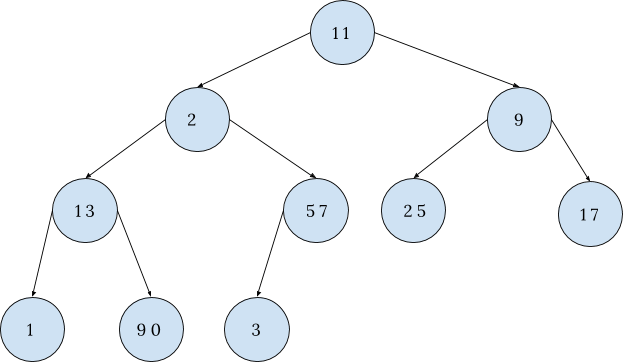11 2 9 13 57 25 17 1 90 3

The above diagram depicts the binary tree version of the array.

We build the heap from the above binary tree and is shown below: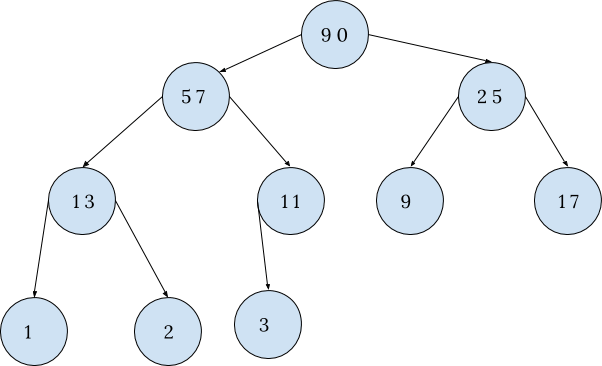90 57 25 13 11 9 17 1 2 3

Now the root 90 is moved to the last location by exchanging it with 3. Finally, 90 is eliminated from the heap by reducing the maximum index value of the array by 1. The balance elements are then rearranged into the heap. The heap and array look like as shown in the below diagram: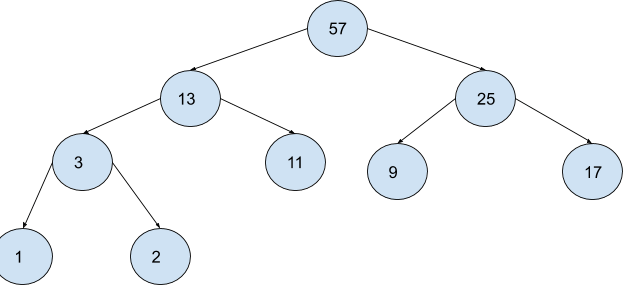57 13 25 3 11 9 17 1 2 90

Similarly when the current root 57 is exchanged with 2, and eliminated from the heap by reducing the maximum index value of the array by 1. The balance elements are then rearranged into the heap. The heap and array look like as shown in the below diagram: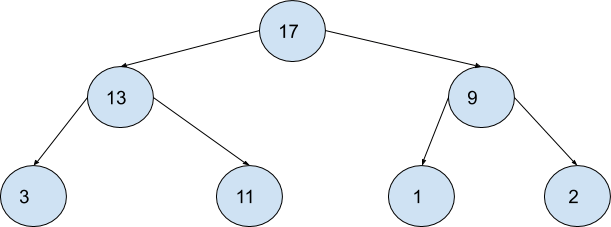17 13 9 3 11 1 2 25 57 90

Following the same approach, the following phases are followed until the fully sorted array is achieved.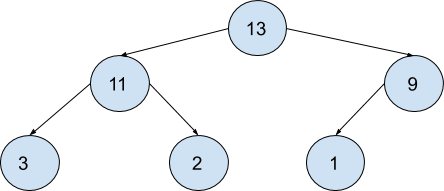13 11 9 3 2 1 17 25 57 90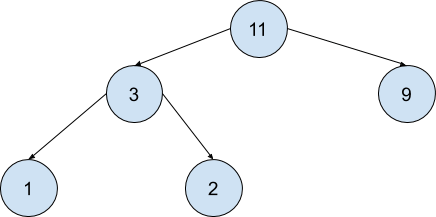11 3 9 1 2 13 17 25 57 90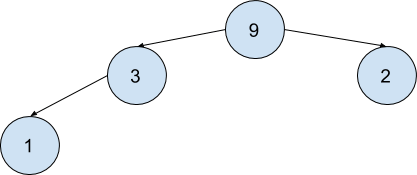9 3 2 1 11 13 17 25 57 90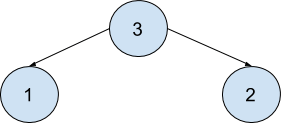3 1 2 9 11 13 17 25 57 90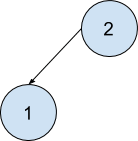2 1 3 9 11 13 17 25 57 90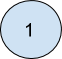1 2 3 9 11 13 17 25 57 90

The array above depicts the fully sorted array using the heap, thus called a heap sort.

### Implementation:

Following are C, C++, Java and Python implementations of Heap Sort.

``````Implementation of heap sort in C:

#include <stdio.h>
int main()
{
int heap, array_size, i, j, c, root, temporary;
printf("\n Enter size of array to be sorted :");
scanf("%d", &array_size);
printf("\n Enter the elements of array : ");
for (i = 0; i < array_size; i++)
scanf("%d", &heap[i]);
for (i = 1; i < array_size; i++)
{
c = i;
do
{
root = (c - 1) / 2;
if (heap[root] < heap[c])   /* to create MAX heap array */
{                                  // if child is greater than parent swap them
temporary = heap[root];      // as structure is of complete binary tree
heap[root] = heap[c];     // it took logn steps to reach from root to leaf
heap[c] = temporary;
}
c = root;
} while (c != 0);
}
printf("Heap array : ");
for (i = 0; i < array_size; i++)
printf("%d\t ", heap[i]);         //printing the heap array
for (j = array_size - 1; j >= 0; j--)
{
temporary = heap;
heap = heap[j] ;   /* swap max element with rightmost leaf element */
heap[j] = temporary;
root = 0;
do
{
c = 2 * root + 1;    /* left node of root element */
if ((heap[c] < heap[c + 1]) && c < j-1)
c++;
if (heap[root]<heap[c] && c<j)    /* again rearrange to max heap array */
{
temporary = heap[root];
heap[root] = heap[c];
heap[c] = temporary;
}
root = c;
} while (c < j);
}
printf("\n The sorted array is : ");
for (i = 0; i < array_size; i++)
printf("\t %d", heap[i]);
}``````
``````Implementation of heap sort in C++:

#include <bits/stdc++.h>
using namespace std;

// To heapify a subtree rooted with node i which is
// Heapify:- A process which helps regaining heap properties in tree after removal
void heapify(int A[], int n, int i)
{
int largest = i; // Initialize largest as root
int left_child = 2 * i + 1; // left = 2*i + 1
int right_child = 2 * i + 2; // right = 2*i + 2

// If left child is larger than root
if (left_child < n && A[left_child] > A[largest])
largest = left_child;

// If right child is larger than largest so far
if (right_child < n && A[right_child] > A[largest])
largest = right_child;

// If largest is not root
if (largest != i) {
swap(A[i], A[largest]);

// Recursively heapify the affected sub-tree
heapify(A, n, largest);
}
}

// main function to do heap sort
void heap_sort(int A[], int n)
{
// Build heap (rearrange array)
for (int i = n / 2 - 1; i >= 0; i--)
heapify(A, n, i);

// One by one extract an element from heap
for (int i = n - 1; i >= 0; i--) {
// Move current root to end
swap(A, A[i]);

// call max heapify on the reduced heap
heapify(A, i, 0);
}
}

/* A  function to print sorted Array */
void printArray(int A[], int n)
{
for (int i = 0; i < n; ++i)
cout << A[i] << " ";
cout << "\n";
}

// Driver program
int main()
{
int A[] = { 22, 19, 3, 25, 26, 7 }; // array to be sorted
int n = sizeof(A) / sizeof(A); // n is size of array

heap_sort(A, n);

cout << "Sorted array is \n";
printArray(A, n);
}``````
``````Implementation of heap sort in Java:

public class heap_sort
{
public void sort(int A[])
{
int n = A.length;

// Build heap (rearrange array)
for (int i = n / 2 - 1; i >= 0; i--)
heapify(A, n, i);   // To heapify a subtree rooted with node i which is
// Heapify:- A process which helps regaining heap properties

// One by one extract an element from heap
for (int i=n-1; i>=0; i--)
{
// Move current root to end
int temporary = A;
A = A[i];
A[i] = temporary;

// call max heapify on the reduced heap
heapify(A, i, 0);
}
}

// To heapify a subtree rooted with node i which is
// an index in arr[]. n is size of heap
void heapify(int A[], int n, int i)
{
int largest = i; // Initialize largest as root
int left_child = 2*i + 1; // left = 2*i + 1
int right_child = 2*i + 2; // right = 2*i + 2

// If left child is larger than root
if (left_child < n && A[left_child] > A[largest])
largest = left_child;

// If right child is larger than largest so far
if (right_child < n && A[right_child] > A[largest])
largest = right_child;

// If largest is not root
if (largest != i)
{
int swap = A[i];
A[i] = A[largest];
A[largest] = swap;

// Recursively heapify the affected sub-tree
heapify(A, n, largest);
}
}

/* A utility function to print array of size n */
static void print_array(int A[])
{
int n = A.length;
for (int i=0; i<n; ++i)
System.out.print(A[i]+" ");
System.out.println();
}

public static void main(String args[])
{
int A[] = {22, 21, 3, 25, 26, 7};
int n = A.length;

heap_sort ob = new heap_sort();
ob.sort(A);

System.out.println("Sorted array is");
print_array(A);
}
}``````
``````Implementation of heap sort in python:
def heapsort(A):
build_max_heap(A)
for i in range(len(A) - 1, 0, -1):
A, A[i] = A[i], A
max_heapify(A, index=0, size=i)
def parent(i):
return (i - 1)//2
def left(i):
return 2*i + 1
def right(i):
return 2*i + 2
def build_max_heap(A):
length = len(A)
start = parent(length - 1)
while start >= 0:
max_heapify(A, index=start, size=length)
start = start - 1
def max_heapify(A, index, size):
left_child = left(index)
right_child = right(index)
if (left_child < size and A[left_child] > A[index]):
largest = left_child
else:
largest = index
if (right_child < size and A[right_child] > A[largest]):
largest = right_child;
if (largest != index):
A[largest], A[index] = A[index], A[largest]
max_heapify(A, largest, size)
A = input('Enter the list of numbers: ').split()
A = [int(x) for x in A]
heapsort(A)
print('Sorted list: ', end='')
print(A)``````

## Serious about Learning Programming ?

Learn this and a lot more with Scaler Academy's industry vetted curriculum which covers Data Structures & Algorithms in depth.

## Heaps And Maps Problems

Heap
Map
Problem Score Companies Time Status
Distinct Numbers in Window 600 39:07
LRU Cache 1000 75:35Free Mock Assessment
Help us know you better for the best experience
Current Employer *
Enter company name